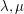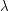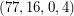Importance: High ✭✭✭
 Author(s):
 Subject: Graph Theory » Algebraic Graph Theory
 Keywords: strongly regular triangle free
 Posted by: mdevos on: May 28th, 2007
Problem   Is there an eighth triangle free strongly regular graph?

A regular graphis strongly regular if there exist integersso that every pair of adjacent vertices have exactlycommon neighbors, and every pair of nonadjacent vertices have exactlycommon neighbors. To eliminate degeneracies, we shall further assume that. Ifis-regular and, then we say thatis astrongly regular graph.

There are exactly seven triangle-free strongly regular graphs known: The five cycle, the Petersen Graph, The Clebsch Graph, the Hoffman-Singleton Graph, The Gewirtz Graph, the Higman-Sims Graph, and astrongly regular subgraph of the Higman-Sims graph. Every Moore Graph of diameter 2 is a triangle-free strongly regular graph, so if there is a 57-regular Moore Graph of diameter 2, this would add another to the list.

See Andries Brouwer's graph descriptions for more on these graphs.

## Bibliography

[G] C. D. Godsil, Problems in Algebraic Combinatorics, Electronic Journal of Combinatorics, Volume 2, F1

* indicates original appearance(s) of problem.